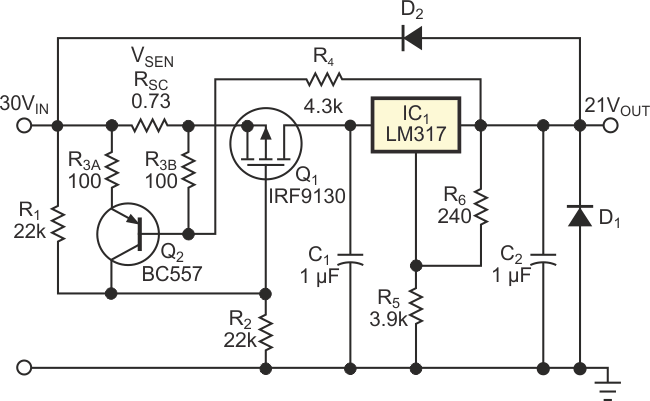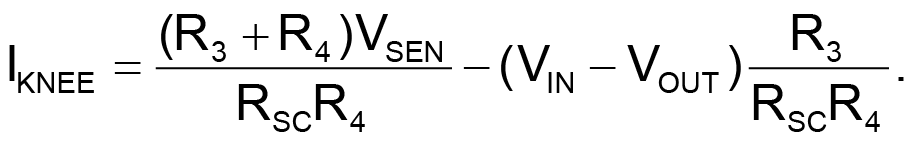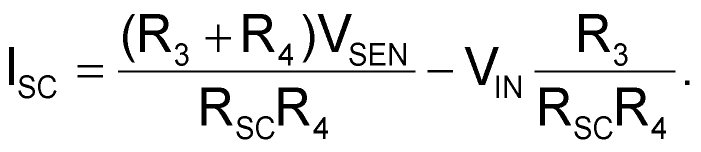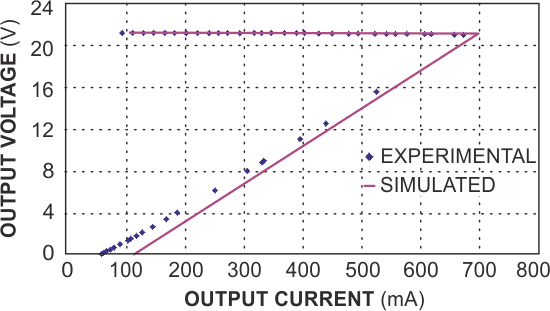## Fairchild LM317

,

For many applications that require power-supply currents of a few amperes or less, three-terminal adjustable-output linear voltage regulators, such as LM317, offer ease of use, low cost, and full on-chip overload protection. The addition of a few components can provide a three-terminal regulator with high-speed short-circuit current limiting for improved reliability. The current limiter protects the regulator from damage by holding the maximum output current at a constant level, IMAX, that doesn't damage the regulator (Reference 1). When a fault condition occurs, the power dissipated in the pass transistor equals approximately VIN×IMAX. Designing a regulator to survive an overload requires conservatively rated - and often overdesigned - components unless you can reduce, or fold back, the output current when a fault occurs (Reference 2).

The circuit in Figure 1 incorporates foldback-current limiting to protect the pass transistor by adding feedback resistor R4. Under normal conditions, transistor Q2 doesn't conduct, and resistors R1 and R2 bias MOSFET Q1 into conduction. When an output overload occurs, Q2 conducts, reducing the on-state bias applied to Q1 and thus increasing its drain-source resistance and limiting the current flowing into regulator IC1, an LM317. Adding R4 makes Q2's bias current dependent on the output voltage, VOUT, which decreases under overload conditions.Figure 1. This circuit adds foldback-overcurrent protection to a linear regulator.

For the circuit in Figure 1, you can calculate the maximum foldover and short-circuit currents, IKNEE and ISC, respectively, as follows:(1)(2)Figure 2. Simulated and measured foldback-current responses to a load resistance that varies from 200 to 0.01 Ω show close agreement.

In a practical design, you select values for IKNEE and ISC and equal values for R3A and R3B and then use equations 1 and 2 to calculate resistors RSC and R4. For the circuit in Figure 1, the output's maximum and short-circuit currents are fixed at 0.7 and 0.05 A, respectively. With R3A and R3B set to 100 Ω, solving the equations yields values of 0.73 Ω for RSC and 4.3 kΩ for R4. You can demonstrate the circuit's performance by applying a variable-load resistor that's adjustable from 0 to 200 Ω. As Figure 2 shows, the output's simulated and measured voltage-versus-current characteristics, VOUT and IOUT, respectively, are in close agreement.

## Materials on the topic

EDN